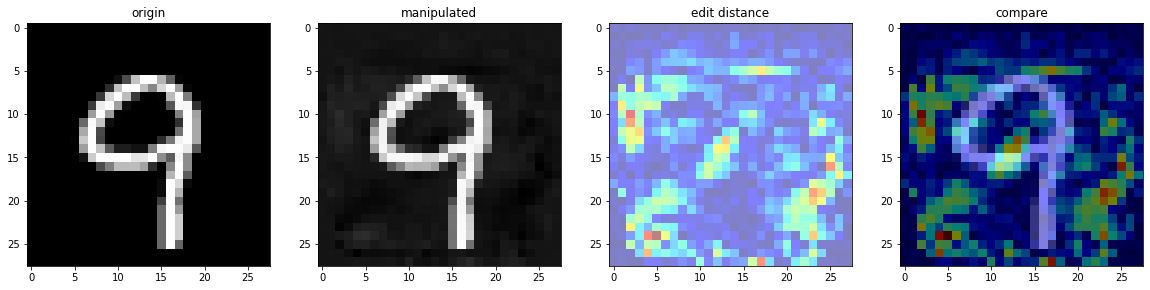首先简要介绍一下欺骗神经网络的原理：

神经网络中几乎到处都有这种机会。例如 sigmoid 函数，自变量在 0 附近的微小改变可以引发函数值很大的变化。一个全连接层，各个节点都变动一个微小数值，没准就能齐心协力把输出的 argmax 改变掉，让分类器作出错误的判断。

CTF 中也经常出现这类「指鹿为马」形式的问题：给出一个分类器（我遇到过神经网络和 k-nearest）的所有参数，然后给出一个样本 $x$，它被模型正确地识别为 $y$. 选手的任务是小幅度地修改 $x$，使得模型将之分类成 $y ^ \prime$. 这里可能要求 $x ^ \prime$ 与 $x$ 的距离小于某个值，例如求出 $x$ 与 $x ^ \prime$ 的每个像素点之间的距离（RGB8，各个通道之差取绝对值求和），要求像素距离总和小于 $10 ^ 5$.

我们之所以可以欺骗神经网络，是因为神经网络缺乏泛化能力。一般来看，过拟合越严重，我们越能轻易地（指 $x ^ \prime$ 与 $x$ 距离很近）修改输入向量，来让神经网络输出我们想要的值。

这是如何做到的？回顾神经网络的训练过程：

1. 在网络中计算 output
2. 求出 output 与 label 的误差，并求出各个网络参数的梯度
3. 利用刚刚求出的梯度，更新网络参数

而我们要欺骗一个已有的神经网络，可以这样做：

1. 在网络中计算 output
2. 求出 output 与我们想要篡改到的 target 的误差，求出图片的梯度
3. 冻结网络的参数，而去对图片进行梯度下降

重复上述过程若干次，可以让神经网络产生误判。

作为演示，我们接下来实现一个神经网络，它识别 MNIST 数据集的手写数字。然后我们随便找一张图像，将其修改一番，使得我们人类仍然能够正确识别，而神经网络给出错误判断。

### 实现神经网络

作为 PyTorch 玩家，我们当然用 PyTorch 来完成此项工作。先把包导入进来：

import torch
import torchvision
import torch.nn as nn
import torchvision.transforms as transforms
import torch.nn.functional as F
import numpy as np

import matplotlib.pyplot as plt

然后导入数据集。 torchvision 库可以帮助我们快速导入 MNIST.

接下来，弄一个训练数据的 loader：

来展示一个数据看看：

x, y = next(iter(train_loader))

plt.imshow(x.squeeze(0), cmap='gray'), y

现在来定义我们的网络。它接收 $28 \times 28$ 的图像，将其摊平成 $784$ 个特征维度的向量。经过一个全连接层，生成 $100$ 维度的特征，激活函数为 ReLU；再经过一个全连接层，生成 $10$ 维度的预测向量，激活函数为 Sigmoid.

接下来生成一个神经网络实例：

定义损失函数和优化器：

现在来训练网络。

开始训练：

fit(net, epoch=5)

'''
epoch 0
[100 / 600] loss=1.512832807302475
test acc: 0.9493999481201172
[200 / 600] loss=1.5147511053085327
test acc: 0.9512999653816223
[300 / 600] loss=1.5131039583683015
test acc: 0.9527999758720398
[400 / 600] loss=1.5105569410324096
test acc: 0.9528999924659729
[500 / 600] loss=1.5110788369178771
test acc: 0.9526000022888184
[600 / 600] loss=1.5110698103904725
test acc: 0.9556999802589417

...

epoch 4
[100 / 600] loss=1.49038764834404
test acc: 0.9696999788284302
[200 / 600] loss=1.4929101729393006
test acc: 0.9692999720573425
[300 / 600] loss=1.490309545993805
test acc: 0.9702999591827393
[400 / 600] loss=1.4926683104038239
test acc: 0.9710999727249146
[500 / 600] loss=1.4924321293830871
test acc: 0.9702000021934509
[600 / 600] loss=1.488825489282608
test acc: 0.9722999930381775
'''

我们这个双层感知机取得了 97.23% 的准确率。接下来我们攻击之。

### 欺骗神经网络

首先取个图出来：

origin_img, origin_tag = next(iter(train_loader))
origin_img = origin_img
origin_tag = origin_tag

plt.imshow(origin_img.view(28, 28), cmap='gray'), origin_tag

现在我们想欺骗神经网络，让网络把 9 误认为 7. 我们冻结网络参数，让神经网络进行反向传播，最后对图片进行梯度下降：

def play(epoch):
for num_epoch in range(epoch):
net.requires_grad_(False)
img.requires_grad_(True)

loss_fn = nn.CrossEntropyLoss()

output = net(img)
target = torch.tensor().to(device)
loss = loss_fn(output, target)

loss.backward()
img.data.sub_(img.grad * .05)
img.grad.zero_()

net.requires_grad_(True)

if num_epoch % 100 == 99:
print(f'[{num_epoch + 1} / {epoch}] loss: {loss} pred: {torch.max(output, 1).item()}')

if torch.max(output, 1).item() == 7:
print(f'done in round {num_epoch + 1}')
return

img = origin_img.detach().to(device).view(1, 28, 28)
img.requires_grad_(True)

play(1000)

'''
[100 / 1000] loss: 2.4601898193359375 pred: 9
[200 / 1000] loss: 1.6000720262527466 pred: 9
done in round 207
'''

我们发现，在迭代 207 次之后，神经网络就把图片误认为是 7 了。来看一下原图和新图的对比：▲ 左起：原图、生成的图、像素差异（颜色越暖差异越大）、像素差异与原图叠加

人眼能轻易地识别为 9，但我们的网络判断这图是 7，于是我们成功地欺骗了神经网络。最后，来欣赏一张关于神经网络泛化能力的漫画：图片来源：reddit，r/ProgrammerHumor